Select Page

# Maths Probability CBSE 12 Science MCQ in English Solutions

Maths Probability CBSE 12 Science MCQ in English Solutions to enable students to get Solutions in a narrative video format for the specific question.

Expert Teacher provides Maths Probability CBSE 12 Science MCQ English Solutions. This video solution will be useful for students to understand how to write an answer in exam in order to score more marks. This teacher uses a narrative style for a question from Probability not only to explain the proper method of answering question, but deriving right answer too.

Please find the question below and view the Solution in a narrative video format.

Question:

Solution Video in English:

You can select video Solutions from other languages also. Please check Solutions in ( Hindi )

## Similar Questions from CBSE, 12th Science, Maths, Probability

Question 1 : If A and B are two events such that P(A)=0.3, P(B)=0.6 and P(B/A)=0.5, find P(A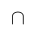B). (View Answer Video)

Question 2 : Evaluate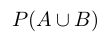, if 2P(A)=P(B)=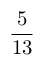and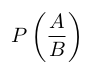=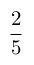. (View Answer Video)

Question 3 : If E and F be two evets such that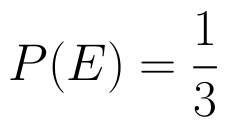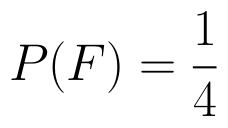, find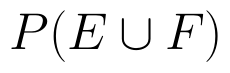if E and F are independent events. (View Answer Video)

Question 4 :  A and B throw a pair of dice alternatively, till one of them gets a total of 10 and wins the game. Find their respective probabilities of winning, if A starts first.   (View Answer Video)

Question 5 :  A man is known to speak the truth 3 out of 5 times. He throws a die and reports that it is 1. Find the probability that it is actually 1.   (View Answer Video)

### Three Dimensional Geometry

Question 1 : Write the distance between the parallel planes 2x - y + 3z = 4 and 2x - y + 3z = 18. (View Answer Video)

Question 2 : Find the vector equation of the line passing through the point (1, 2, 3) and parallel to the planes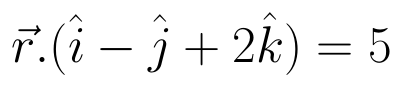and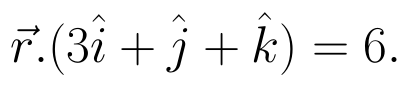(View Answer Video)

Question 3 : Find the distance of the point (1, -2, 3) from the plane x-y+z=5 measured parallel to the line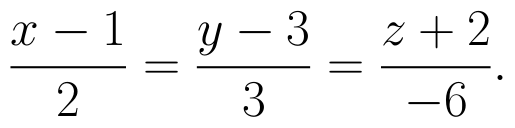(View Answer Video)

Question 4 : Find the distance of point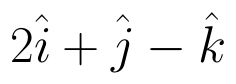from the  plane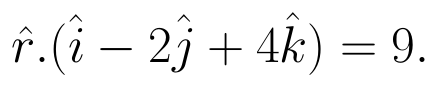(View Answer Video)

Question 5 : Find the distance between the point (-1, -5, -10) and the point of intersection of line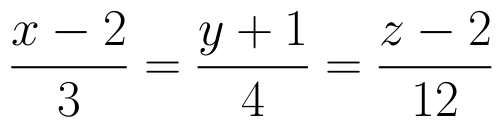and plane

### Application of Integrals

Question 1 : Using integration, find the area of the triangle formed by a positive x-axis and tangent and normal to the circle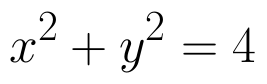at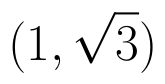. (View Answer Video)

Question 2 : Using integration find the area of the triangular region whose sides have the equations y = 2x + 1, y = 3x + 1 and x = 4. (View Answer Video)

Question 3 : Using the method of integration, find the area of the region bounded by the lines 3x - y - 3 = 0, 2x + y - 12 = 0 and x -2y - 1 = 0. (View Answer Video)

Question 4 : Area lying in the first quadrant and bounded by the circle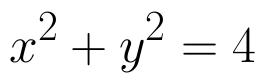and the lines x =0 and x = 2 is (View Answer Video)

Question 5 : A farmer has a field of shape bounded by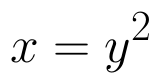and x = 3, he wants to divide this into his two sons equally by a straight line x = c. Can you find c? What value, you find in the person ? (View Answer Video)

### Integrals

Question 1 : Let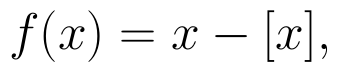for every real number, x, where [x] is the greatest integer less than or equal to x.
Evaluate :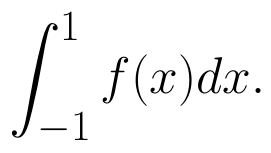(View Answer Video)

Question 2 : Prove that :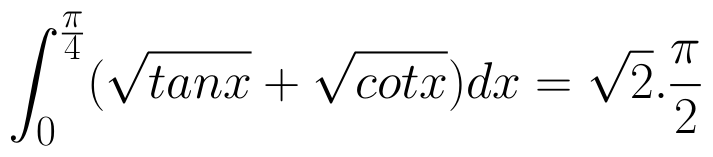(View Answer Video)

Question 3 : Find: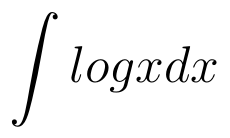. (View Answer Video)

Question 4 : Show that :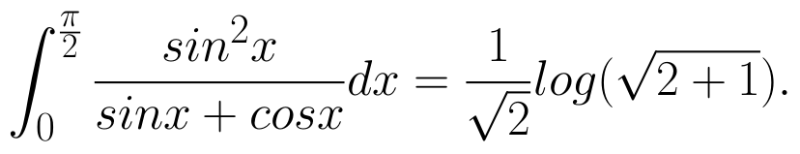(View Answer Video)

Question 5 : Evaluate :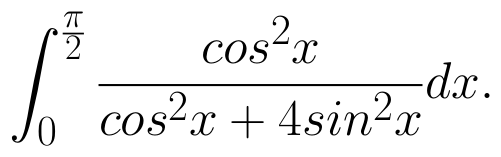(View Answer Video)